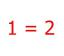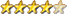3 phase inverter for AC induction and servo motors

Author Message
poidaGuruJoined: 02/02/2017
Location: Australia
Posts: 1114
 Posted: 12:14am 05 Jan 2021

Simple VFD 3 phase motor drives use a control principle that
keeps the Voltage and frequency ratio constant.
Let's say the drive is set to output 240V AC at 50 Hz.
This ratio is 4.8
The output voltage will need to be altered to maintain this ratio
as the frequency changes resulting from the operator's input.

eg. A freq of 25Hz means 1/2 the 240V or 120V. (120/25 = 4.8)

Looking at some important facts of centrifugal water pumps
from here gives
flow rate is proportional to RPM
head or output pressure proportional to RPM squared
motor power is proportional to RPM cubed

So for a doubling of RPM, the motor will need 8 times the power.
And for 1/2 RPM, the motor now needs 1/8th.
So RPM of the motor is a powerful controlling factor of the power flowing through the drive when driving a cf water pump.

I am looking at the outline of the control scheme for the solar powered 3 phase water pump and it's time to flesh out a few things.

The solar array would need to be wired to give something like 60V at maximum power point. The motor is 2 hp or 1500W. A power level for the motor when running at 25Hz is measured during system build. And the power level at 50 Hz is measured.

The inverter will need DC supply voltage and current sensing.
The primary winding peak to peak voltage should be about 80% of the 60V supply.
0.8 x 60 X 0.707 = 34V AC RMS primary for 240V AC RMS on the secondary output.

IF motor not running:

The firmware would periodically check if the array voltage is greater than
some reasonable start value. IF greater, then soft start motor to 25Hz.
Only if input DC power = correct already known power level FOR 25Hz will it keep running. Else it soft stops.

IF motor running:

periodically increase output frequency a small amount and check
if DC input power increases as expected. IF the input power drops, reduce
the frequency a small amount. Only permit frequency to reach 50Hz.
Also, if frequency needs to drop below 25Hz due to insufficient DC input
power, stop motor.

The search for fastest RPM or frequency while keeping input power adequate
is similar to MPPT tracking. And always modify output voltage in keeping with the
required V/f ratio of 4.8.

The 50Hz to 25Hz range gives 1500W to 188W operating range for input power.
It gives a factor of 2 flow rate range.
I presume the pump will be sized such that at 25Hz it can pump water up the
rise of the bore hole easily. Recall the frequency squared dependence for head pressure.

I think this will work fine without any need for a battery.

The build:
One and one half inverter power boards, 3 primary chokes, 3 toroids, 3 12V output Vfb transformers, a current sensor and the control board based on the Arduino Mega.

Why is there a need for constant V/f ratio?

My view is the motor is an inductor and so if a voltage is applied for long enough
then the inductor will saturate. From our inductor testing we found
L = V x s / I
where L = inductance (Henries), s = time (seconds) and I = current (Amps)
From the above it's easy to see the V x s part, and if the voltage and time
stay at a constant ratio, we will have no problems with increasing current.
(the inductance stays the same)

I just verified this with the single phase inverter on the bench.
I have a modified firmware which permits changing output frequency from 40
to 60Hz. The toroid starts to saturate at about 45Hz with my 240V output.
Saturation increases as the frequency drops further.

A change to the code to have output voltage drop as the frequency drops
now prevents saturation. I maintain the voltage/frequency ratio as a constant
over the 40 to 60 Hz.

I suspect the induction motor behaves the same way